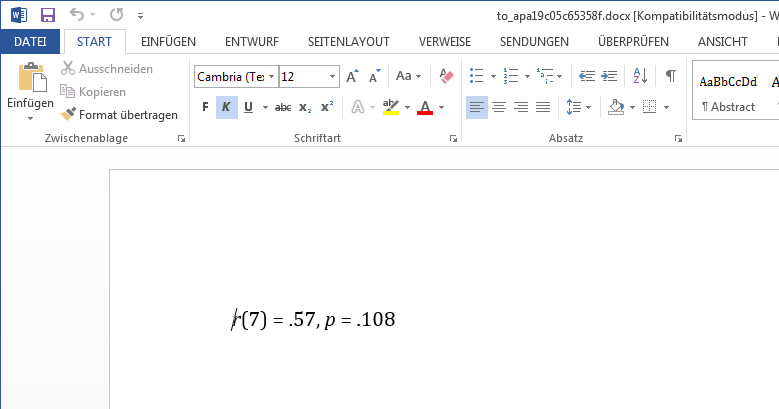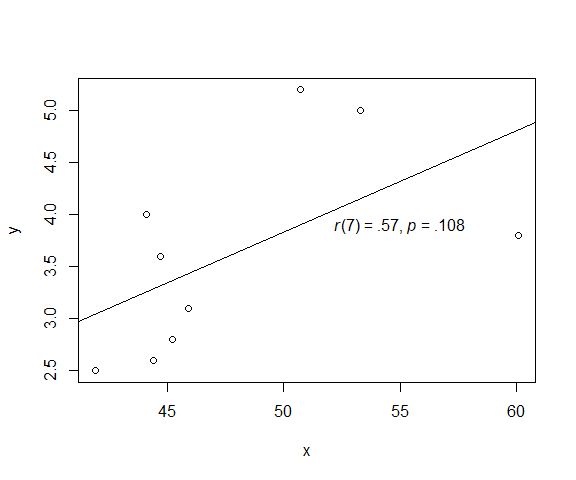# Introduction to apa

#### 2019-01-07

The *_apa() functions help you to format outputs of statistical tests according to guidelines of the APA (American Psychological Association).

The functions take the return value of a test function as the first argument, e.g. a call to chisq.test() is passed to chisq_apa(), which returns a formatted string.

The idea of such formatters was introduced in the schoRsch package. apa generalizes this idea by providing formatters for different output formats (text, Markdown, RMarkdown, HTML, LaTeX, LaTeX inline math, docx and R’s plotmath syntax).

Currently supported tests are:

• t-test (t.test and apa::t_test)
• ANOVA (aov, ez::ezANOVA, afex::aov_car, afex::aov_ez, and afex::aov_4)
• chi-squared test (chisq.test)
• test of a correlation (cor.test)

## Example

Take the following test of a correlation as an example:

# Data from ?cor.test
x <- c(44.4, 45.9, 41.9, 53.3, 44.7, 44.1, 50.7, 45.2, 60.1)
y <- c( 2.6,  3.1,  2.5,  5.0,  3.6,  4.0,  5.2,  2.8,  3.8)

ct <- cor.test(x, y)
ct
##
##  Pearson's product-moment correlation
##
## data:  x and y
## t = 1.8411, df = 7, p-value = 0.1082
## alternative hypothesis: true correlation is not equal to 0
## 95 percent confidence interval:
##  -0.1497426  0.8955795
## sample estimates:
##       cor
## 0.5711816

Calling cor_apa() then returns a string ready to copy-and-paste into manuscripts or presentations.

cor_apa(ct)
## r(7) = .57, p = .108

The format argument of cor_apa() allows you to specify the output format, which can be one of "text" (default), "markdown", "rmarkdown", "html", "latex", "latex_math", "docx" or "plotmath".

cor_apa(ct, format = "rmarkdown")
## *r*(7) = .57, *p* = .108

Which is printed as r(7) = .57, p = .108 in a RMarkdown document.

cor_apa(ct, format = "latex")
## \textit{r}(7)~=~.57, \textit{p}~=~.108
# Opens a temporary document in your word processor
cor_apa(ct, format = "docx")# Paste output in a plot using R's plotmath syntax
plot(x, y)
abline(lm(y ~ x))
text(55, 3.9, cor_apa(ct, format = "plotmath"))